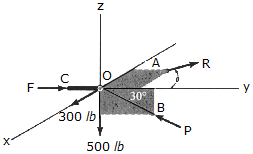# Engineering Mechanics - Equilibrium of a Particle - Discussion

### Discussion :: Equilibrium of a Particle - General Questions (Q.No.1)

1.The joint O of a space frame is subjected to four forces. Strut OA lies in the x-y plane and strut OB lies in the y-z plane. Determine the force acting in each if the three struts required for equilibrium of the joint. Set= 45°.

 [A]. F = 46.4 lb, R = 400 lb, P = 424 lb [B]. F = 566 lb, R = 424 lb, P = 1000 lb [C]. F = 11.3 lb, R = 424 lb, P = 577 lb [D]. F = 1166 lb, R = 424 lb, P = 1000 lb

Explanation:

No answer description available for this question.

 Rajudev said: (Mar 30, 2011) I can't understand.

 Nikhil said: (Jun 17, 2011) How to solve?

 Dilip said: (Jul 30, 2011) How to find angle for 300?

 Aditya said: (Aug 3, 2011) Sum of force in z direction -500 + p sin30 = 0 p=1000 sum of force in x direction 300 - r sin45 =0 r = 424.26 sum of force in y direction p cos30 - r cos45 = f f = 566.025

 James Turner said: (Aug 10, 2011) How did you come up with the value of p ?

 Vasu said: (Aug 27, 2011) Put sin30 value and calculate.

 Ajinkya said: (Sep 28, 2011) Good calculation aditya. Thanks.

 Srikanth said: (Oct 20, 2011) Good work aditya thanks.

 Afiq said: (Feb 12, 2012) How to convert lb to newton?

 Sanatan Singh said: (Mar 2, 2012) Why to convert the unit from lb to newton (N) when the answer required is in lb ? Simple question just based on laws of static equilibrium. Ex=0 Ey=0 Ez=0

 Bikash said: (Mar 7, 2012) Sum of forces in a direction =0 taking E Fx=0; E Fy=0; E Fz=0; Solve it simple.

 K.Vishnu said: (Nov 23, 2012) 300=R*sin45 R=424 500=P*sin30 P=1000 And F=P*cos30-R*cos45 F=1000*Sqrt3/2-424/sqrt2 F=566

 Santosh Kumar Sahu said: (Apr 1, 2016) Good @Aditya, nice.

 Chitti said: (Dec 15, 2016) Good job @Aditya.

 Hari said: (Sep 20, 2017) Thanks @Aditya.

 Raja said: (Nov 25, 2017) Thanks @Aditya.

 Patience said: (May 17, 2018) Thank you for the explanation.

 Narendra said: (Jul 13, 2021) How to solve it? Please explain.